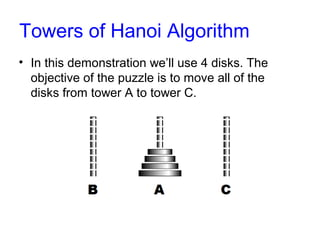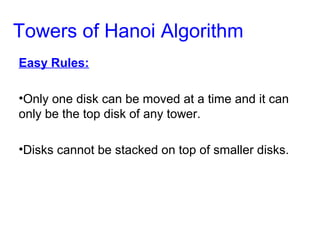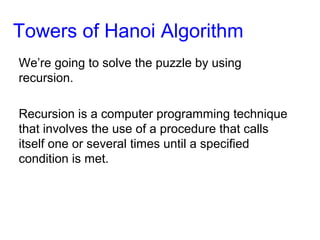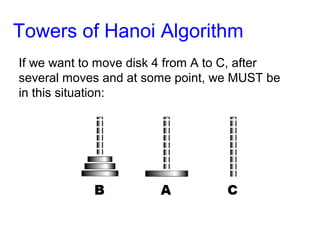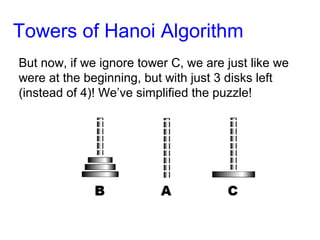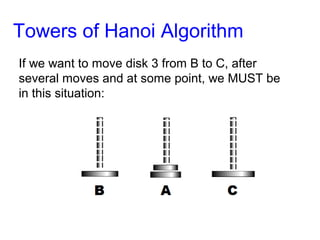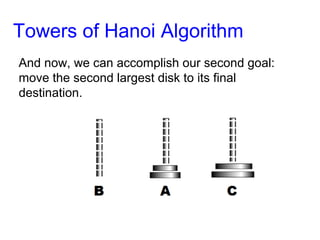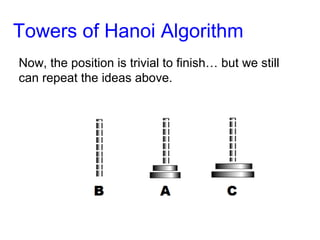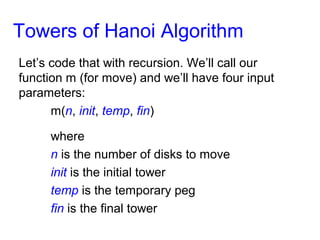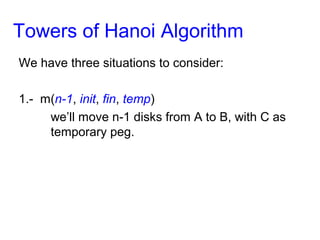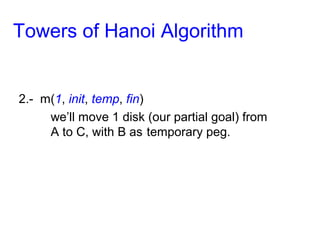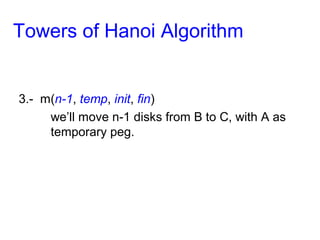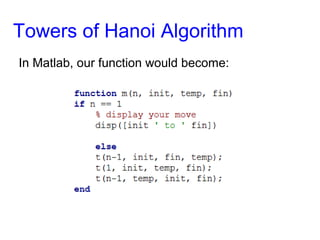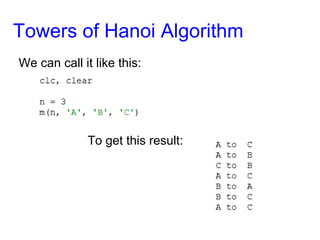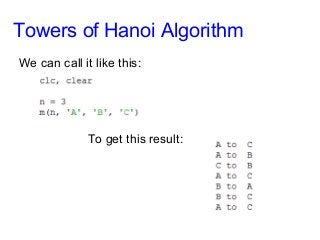1 of 19

### Towers Hanoi Algorithm

1. The Towers of Hanoi Algorithm In Matlab
2. • In this demonstration we’ll use 4 disks. The objective of the puzzle is to move all of the disks from tower A to tower C. Towers of Hanoi Algorithm
3. Towers of Hanoi Algorithm Two Easy Rules: •Only one disk can be moved at a time and it can only be the top disk of any tower. •Disks cannot be stacked on top of smaller disks.
4. Towers of Hanoi Algorithm We’re going to solve the puzzle by using recursion. Recursion is a computer programming technique that involves the use of a procedure that calls itself one or several times until a specified condition is met.
5. Towers of Hanoi Algorithm If we want to move disk 4 from A to C, after several moves and at some point, we MUST be in this situation:
6. Towers of Hanoi Algorithm And now, we can accomplish our first goal: move the largest disk to its final destination.
7. Towers of Hanoi Algorithm And now, we can accomplish our first goal: move the largest disk to its final destination.
8. Towers of Hanoi Algorithm But now, if we ignore tower C, we are just like we were at the beginning, but with just 3 disks left (instead of 4)! We’ve simplified the puzzle!
9. Towers of Hanoi Algorithm If we want to move disk 3 from B to C, after several moves and at some point, we MUST be in this situation:
10. Towers of Hanoi Algorithm And now, we can accomplish our second goal: move the second largest disk to its final destination.
11. Towers of Hanoi Algorithm And now, we can accomplish our second goal: move the second largest disk to its final destination.
12. Towers of Hanoi Algorithm Now, the position is trivial to finish… but we still can repeat the ideas above.
13. Towers of Hanoi Algorithm Let’s code that with recursion. We’ll call our function m (for move) and we’ll have four input parameters: m(n, init, temp, fin) where n is the number of disks to move init is the initial tower temp is the temporary peg fin is the final tower
14. Towers of Hanoi Algorithm We have three situations to consider: 1.- m(n-1, init, fin, temp) we’ll move n-1 disks from A to B, with C as temporary peg.
15. Towers of Hanoi Algorithm 2.- m(1, init, temp, fin) we’ll move 1 disk (our partial goal) from A to C, with B as temporary peg.
16. Towers of Hanoi Algorithm 3.- m(n-1, temp, init, fin) we’ll move n-1 disks from B to C, with A as temporary peg.
17. Towers of Hanoi Algorithm In Matlab, our function would become:
18. Towers of Hanoi Algorithm We can call it like this: To get this result:
19. Towers of Hanoi Algorithm For more examples and details, visit: matrixlab-examples.com/tower-of-hanoi-algorithm.html